# CAHSEE - Quadratics & Polynomials: Help and Review Chapter Exam

Exam Instructions:

Choose your answers to the questions and click 'Next' to see the next set of questions. You can skip questions if you would like and come back to them later with the yellow "Go To First Skipped Question" button. When you have completed the practice exam, a green submit button will appear. Click it to see your results. Good luck!

### Page 1

#### Question 1 1. Divide the following polynomials: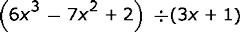#### Question 2 2. Divide using long division.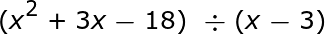#### Question 3 3. Find the value for c and rewrite the equation in the vertex form.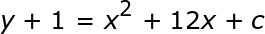#### Question 4 4. Divide the following polynomials: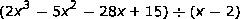### Page 2

#### Question 8 8. Solve the equation below.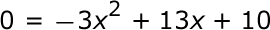### Page 3

#### Question 12 12. Use the quadratic formula to find the solutions to the equation below.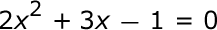#### Question 14 14. Solve the following inequality.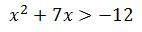### Page 4

#### Question 16 16. Divide the following polynomials: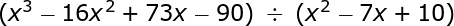#### Question 17 17. Expressing the equation below in the vertex form will give a value of 'h' = _____ .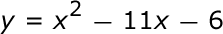#### Question 18 18. Rewrite the quadratic below in standard form: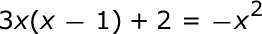#### Question 19 19. Find the roots of the equation below.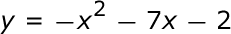#### Question 20 20. Which statement is true about the inequality below?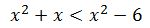### Page 5

#### Question 23 23. When completing the square on the quadratic equation listed below, how is c calculated?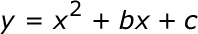### Page 6

#### Question 28 28. If the equation below told you a ball was 'h' feet in the air after 't' seconds, what would be the first step to finding out when it hit the ground?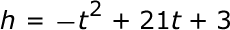#### Question 29 29. What is the b-value of the quadratic equation below?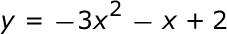#### Question 30 30. Solve the equation.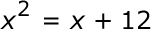#### CAHSEE - Quadratics & Polynomials: Help and Review Chapter Exam Instructions

Choose your answers to the questions and click 'Next' to see the next set of questions. You can skip questions if you would like and come back to them later with the yellow "Go To First Skipped Question" button. When you have completed the practice exam, a green submit button will appear. Click it to see your results. Good luck!

Support